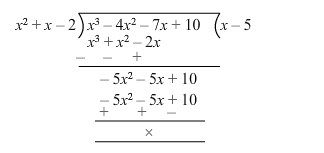# If 1 and −2 are two zeros of the polynomialQuestion:

If 1 and $-2$ are two zeros of the polynomial $\left(x^{3}-4 x^{2}-7 x+10\right)$, find its third zero.

Solution:

Let $f(x)=x^{3}-4 x^{2}-7 x+10$

Since 1 and $-2$ are the zeroes of $f(x)$, it follows that each one of $(x-1)$ and

$(x+2)$ is a factor of $f(x)$

Consequently, $(x-1)(x+2)=\left(x^{2}+x-2\right)$ is a factor of $f(x)$.

On dividing $f(x)$ by $\left(x^{2}+x-2\right)$, we get:$f(x)=0=>\left(x^{2}+x-2\right)(x-5)=0$

$=>(x-1)(x+2)(x-5)=0$

$\Rightarrow x=1$ or $x=-2$ or $x=5$

Hence, the third zero is 5 .